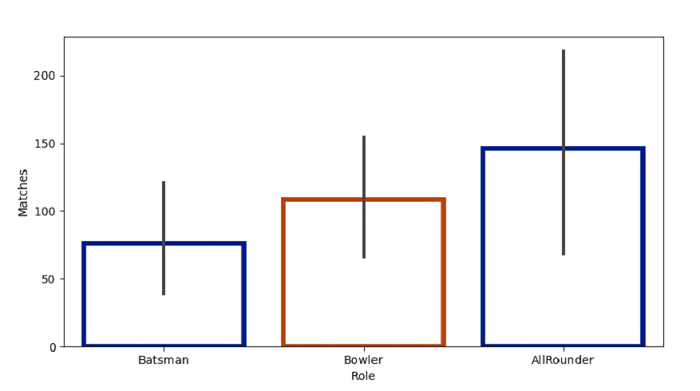# Python Pandas - Create a Bar Plot and style the bars in Seaborn

Bar Plot in Seaborn is used to show point estimates and confidence intervals as rectangular bars. The seaborn.barplot() is used. Style the bars using the facecolor, linewidth and edgecolor parameters.

Let’s say the following is our dataset in the form of a CSV file −Cricketers2.csv

At first, import the required libraries −

import seaborn as sb
import pandas as pd
import matplotlib.pyplot as plt

Load data from a CSV file into a Pandas DataFrame −

dataFrame = pd.read_csv("C:\Users\amit_\Desktop\Cricketers2.csv")


Design the bars −

sb.barplot(x=dataFrame["Role"], y=dataFrame["Matches"], facecolor=(1, 1, 0, 0), linewidth=4, edgecolor=sb.color_palette("dark", 2))

## Example

Following is the code −

import seaborn as sb
import pandas as pd
import matplotlib.pyplot as plt

# Load data from a CSV file into a Pandas DataFrame

# plotting bar plot with Role and Matches column
# designing the bars
sb.barplot(x=dataFrame["Role"], y=dataFrame["Matches"], facecolor=(1, 1, 0, 0), linewidth=4, edgecolor=sb.color_palette("dark", 2))

# display
plt.show()



## Output

This will produce the following output −